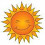## Wednesday, January 12, 2011

### Joe Newbury

Tucson massacre / VPI strangeness

1.Her wiki page did discuss the tattoo. But no longer.

http://www.highbeam.com/doc/1P1-89529173.html

2.^^^^^^^^^^^^^^^^^^^^^^^^^^^^^^^^^^^^^^^^^^^^^
^^^^^^^^^^^^^^^^^^^0^^^^^^^^^^^^^^^^^^^^^^^^^

fellist wrote:

.......it is hard to imagine that the poetry or prose of either Newbury.....

OMG Newbury writes "poetry"??!! LOL

How many times does the word "slope head" appear in his "poems"???!!

Newbury writes "poetry"??!! LOL

^^^^^^^^^^^^^^^^^^^0^^^^^^^^^^^^^^^^^^^^^^^^^
^^^^^^^^^^^^^^^^^^^^^^^^^^^^^^^^^^^^^^^^^^^^^

3.The involvement of the authorities with Newbury, and the similar role played by the Chair of the English Department with both Cho and Newbury, suggest some kind of law enforcement experiment in psychological profiling was involved with both. The experiment went sideways with tragic results if true.